Application

# How to Know the Parameters of the Accelerometer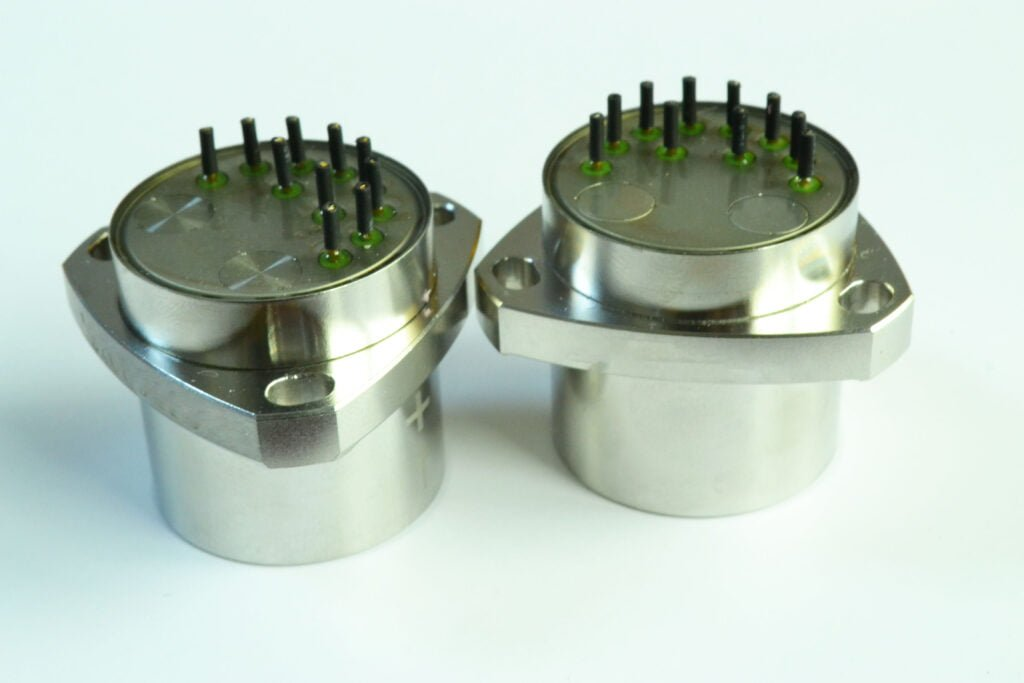The main parameters of the accelerometer

Taking ERICCO‘s quartz accelerometer ER-QA-03A as an example, the meaning of the main parameters is briefly described.

Measurement Range

Refers to the acceleration range that the accelerometer can measure. The larger the value, the stronger the ability to measure the magnitude of acceleration. The measurement range should be reasonably selected for different applications. Because the measurement range is large, the sensitivity will be correspondingly reduced, unless a higher precision is selected.

For the ER-QA-03A series, the measurement range of ER-QA-03A1 ER-QA-03A2 ER-QA-03A3 is ±50g.

Bias

It specifies the device output when the measurement axis acceleration is 0g. It can be given as a voltage value (analog output device), or in LSB (digital output device), or in mg. It can be understood as a static error that does not fluctuate over time. The user can directly subtract 0g Bias from the measured value for compensation. For example ER-QA-03A1 bias is ≤ (±3)mg.

There are usually several representations:

Deviation from ideal value at 25°C

The change of 0g offset with temperature, that is, how many mg of output change for every 1°C change

Total offset including all errors

Which representation method is used depends on the application market defined by the chip and what its traditional definition method is.

Noise (NOISE)

The power spectral density of the noise output is generally given in the form of µg/√Hz. The higher the bandwidth, the greater the noise. The bandwidth is roughly equal to half of the output data rate. Therefore, the higher the output rate is set, the greater the noise.

The total noise is determined by the noise density and bandwidth (BW): total noise=noise density*SQRT(1.6*BW). The appropriate bandwidth should be selected for different applications to reduce the total noise. Usually the various movements of people are at 50 to 100 Hz; the tilt detection is about 5 Hz; the impact detection is >1 kHz. The characteristics of the noise determine the accuracy of the device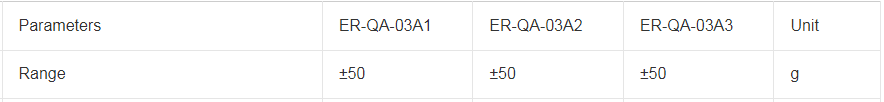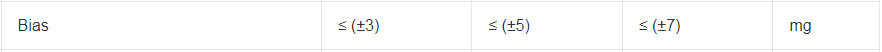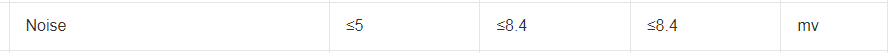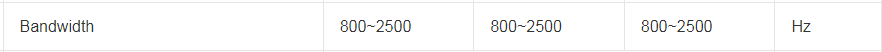OUTPUT DATA RATE AND BANDWIDTH

The output data rate (ODR) refers to the amount of information (bits) transmitted on the channel per unit time. The bandwidth is 1/2 of the ODR.